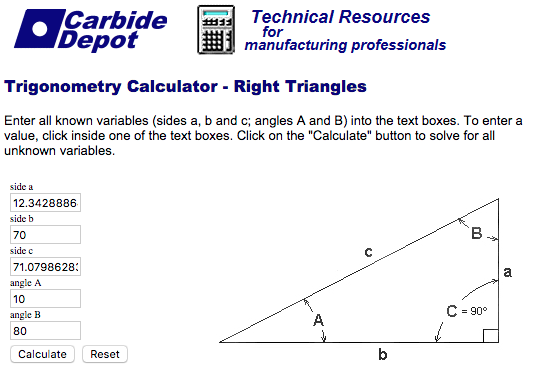# How can I change the angle of fluid flow?

Say I want to change the angle of attack of an airfoil without having to change the angle of the object. How can I change z velocities angle on the velocity inlet?

Hi @glorincz!

For this you just have to use basic trigonometric operations. So for a flow with an angle you would use more than one component of the velocity which will be non-zero. I have posted an illustration of that somewhere in the forum. Let me search it and post it here as soon as I find itCheers!

Jousef

Great, thank you!

1 Like

In this case, can I use the airflow speed as distance? I can calculate the direction if I use the desired angle of attack and the base of the triangle (Ux), in theory. Am I right about that?

Hi @glorincz!

You’re just using the velocities as depicted in the pictures above. Length and speed are different quantities but if you mean the length of the vector then yes, correct. It also depends on how your coordinate system is defined, if you share the project I can see which one the “up-direction” is and this is the second component that you would need (besides the one in the flow direction). Hope that this was not too confusing!

Best,

Jousef

1 Like

Not at all! Thank you! I will try to use airflow speed (m/s) as a vector length.

So yes, the velocity can be used as a vector and managed to change the angle of attack. Here I entered the desired angle of attack (10 degrees, angle A) and the velocity (70m/s, side B). The calculator gives 12.34… (side A) as the desired vector. In Simscale I just added 12.34m/s at Vz.Note how the pressure changes as the profile changes (negative to positive camber). The angle of attack is 10 degrees.